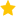See the live dashboard for CensusAtSchool 2023

We explore single numeric variables and learn how to plot and interpret them. We discover how to describe the shape, centre and spread of dot plots and look for oddities This resource contains:

1. Numeric variables (video, 6 min)
2. Features of numeric variables (summarizing explanatory document)
3. Feature spotting (video, 5 min)

Numeric Variables

Plotting numeric variables shows us interesting characteristics of our data. In this video we’ll explore pulse rates and household incomes and learn how to describe what we see.

After you’ve watched these videos, you should be able to answer these questions:

• How is a stacked dot plot constructed?
• What are the four types of things we look for in dot plots?
• What is the common name for the mean?
• How does the mean relate to the dot plot?
• What property defines the median?
• When do the mean and median tend to be very similar, and when can they be quite different?
• Why might we prefer the median to the mean for personal incomes?

Features of numeric variables

Learn to identify and interpret the features of numeric variables that are evident on dot plots, box plots and summary tables.

The linked pdf is both a reading and reference document. It lays out the main ideas relating to Centre, Spread, Shape and Oddities for a numeric variable. It is done in a landscape tabular form designed to make it easier to see the inter-relationships between ideas. It is a core part of the teaching about these issues. The video that follows assumes that the viewer has already read the pdf.

Feature spotting

n this video we’ll introduce you to box plots and measures of spread, and to shape and oddities. We’ll use our knowledge to look at our data, to draw insights from it, and possibly ask more questions about the data. Why are weights bimodal and heights not?

After you’ve watched these videos, you should be able to answer these questions:

• What sort of “oddities” should we look for and what sort of questions should we ask when we see them?
• What is the defining property of a median? the first quartile? and third quartile?
• What information does the box plot add to the dot plot?
• What is the interquartile range and how does it relate to the box plot?
• What is an “outlier”?
• What does “positively skewed” mean?
• What does “bimodal” mean?
• Event: Data to Insight
• Keywords: Bimodal, Box plots, Central tendency, Distribution Shape, Dot Plots, Five Number Summary, Histograms, Interpreting displays, interquartile range, Mean, Median, Outlier, Spread, Unimodal
• (Last updated: 10/11/17. Added: 08/11/17)
• ## Rate this resource:(2 ratings)